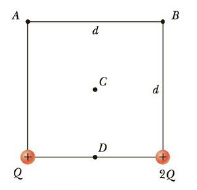Chapter 16, Problem 13CQ

Chapter
Section
Textbook Problem

Rank the electric potentials at the four points shown in Figure CQ16.13 from largest to smallest.Figure CQ16.13

To determine
The order of potential at various points.

Explanation

The potential at A is,

VA=keQd+ke(2Q)2d=keQd(1+2)=2.414keQd

• ke is the Coulomb constant.

The potential at B is,

VB=ke(2Q)d+keQ2d=keQd(2+12)=2

Still sussing out bartleby?

Check out a sample textbook solution.

See a sample solution

The Solution to Your Study Problems

Bartleby provides explanations to thousands of textbook problems written by our experts, many with advanced degrees!

Get Started

Find more solutions based on key concepts